# IBPS RRB 2018 Prelims : Reasoning – Day 15

Dear Bankersdaily Aspirants,

IBPS RRB PO & Office Assistant 2018 Preliminary Examination starts from 11th August, 2018. The IBPS RRB Exam 2018 is one of the most expected exam of the year. The reason for the consummate expectation is different from that of the others.

The number of candidates attending the IBPS RRB PO & Office Assistant is high when compared to the other exams like SBI & Insurance Exams. The other important factor is that , no English section is there in the preliminary examination of the IBPS RRB PO & Assistant Exams. This is really a boost to the candidates as many of the aspirants couldn’t get the requisite marks in the English section and this exam is the best bet for the aspirants.

Since only few days are left for the IBPS RRB PO & Assistant Exam 2018 Preliminary Exam , we will be providing daily 20 Questions in Quantitative Aptitude & Reasoning Section to evoke the preparation mode.

Aspirants can answer the Questions by commenting the answers for the Questions in the comments section. We will be providing the answers for the Questions @ 6:00 P.M everyday.

## IBPS RRB PO & Assistant 2018 – Prelims Exam – Day 15

Topic : Reasoning Ability

Total Number of Questions : 20

Total Time : 15 minutes

D.1-5) In each question, relationship between different elements is shown in the statements. The statements are followed by conclusions. Study the conclusions based on the given statements and select the appropriate answer.

Q.1) Statements:

V=M>J≥R>P; E≤A=J≥N

Conclusions:

I. A>P

II. E<R

III. M>E

IV. R≥N

a) Both II and IV follow

b) Only III follows

c) Both I and III follow

d) Only IV follows

e) None of these

Q.2) Statements:

K=T≤L; K>R≤M; I>N=M; I>J

Conclusions:

I. T<N

II. L>N

III. K>J

IV. L<M

a) Only III follows

b) Both II and III follow

c) Only IV follows

d) Only I follows

e) None follows

Q.3) Statements:

D=M<N; H=I≤D; S=Q<X=N; H>B<F<R

Conclusions:

I. I<Q

II. S>R

III. B>X

IV. B<X

a) Only III follows

b) Both II and III follow

c) Only IV follows

d) Only I follows

e) None follows

Q.4) Statements:

V>U≤B; V=R≤M; I>N<M; B>J<O

Conclusions:

I. U<N

II. B>N

III. V>J

IV. B<M

a) Only III follows

b) Both II and III follow

c) Only IV follows

d) Only I follows

e) None follows

Q.5) Statements:

F<W>Z≥R≥L; E=A>Z≥N.

Conclusions:

I. A>L

II. E<R

III. W=E

IV. R≥N

a) Both II and IV follow

b) Only III follows

c) Both I and II follow

d) Only IV follows

e) None of these

D.6-10) In each question below are given some statements followed by some conclusions. You have to take the given statements to be true even if they seem to be at variance with commonly known facts. Read all the conclusions and then decide which of the given conclusions logically follow from the given statements.

Q.6) Statements:

Some homes are building.

All homes are house.

All buildings are house.

Some houses are apartment.

Conclusions:

I. Some buildings are apartment

II. No apartment is a home

III. All apartments can never be home

a) Only I and II follow

b) Only III follows

c) Only II follows

d) None follows

e) None of these

Q.7) Statements:

No light is a fan.

Some fans are wind.

No wind is a storm.

Conclusions:

I. Some lights are storm

II. All fans can never be storm

III. Some winds are definitely not light

a) Only I and II follow

b) Only III follows

c) Only II and III follow

d) All follow

e) None of these

Q.8) Statements:

Some chairs are table.

No table is a bench.

No bench is a desk.

Conclusions:

I. All tables can never be desk

II. Some chairs are benches

III. No bench is a chair

a) Only I and either II or III follow

b) Only III follows

c) Either II or III follows

d) Only I follows

e) None of these

Q.9) Statements:

All camels are giraffe.

No giraffe is a deer.

All deer are cow.

Conclusions:

I. Some cows are giraffe is a possibility

II. No deer is a camel

III. At least some camels are cow

a) Only I and II follow

b) Only III follows

c) Only II and III follow

d) None follows

e) None of these

Q.10) Statements:

Only tigers are cat.

Some tigers are leopard.

No leopard is a cheetah.

Conclusions:

I. Some tigers are cheetah is a possibility

II. All cats can never be leopard

III. No cheetah is a tiger

a) Only II follows

b) Only I follows

c) Either I or III follows

d) Only II and either I or III follows

e) None of these

D.11-15) Study the following information carefully to answer the given questions.

C # 9 ^ Y 4 K & A 6 N > 0 M 1 E * 3 H 2 L @ I 5 X \$ 8 V 7 % O J

Q.11) In the above sequence how many such pairs are there in each of which numbers are immediately preceded by a symbol and immediately followed by a consonant?

a) One

b) Two

c) More than three

d) Three

e) None

Q.12) If in the above sequence all the numbers are dropped then, which of the following element is sixth to the left of seventh from the right end?

a) E

b) *

c) M

d) N

e) @

Q.13) Four of the following five are alike in a certain way based on the arrangement and hence form a group. Which one of the following does not belong to the group?

a) C#J

b) Y4V

c) A65

d) N>I

e) ^Y7

Q.14) In the following series what should come in place of question mark?

C9Y #^K 9YA ?

a) ^4E

b) ^K>

c) ^49

d) ^4M

e) None of these

Q.15) If all the vowels are coded as its second previous alphabetic letter, and all the consonants are coded as its third next alphabetic letter. After coding how many vowels are there in the arrangement?

a) One

b) Two

c) Four

d) Three

e) None

D.16-17) Study the following information carefully and answer the questions given below.

A, B, C, D, E and F are the six members of a family and there are two married couple in the family. A is the father of E. C is the sister-in-law of G who does not have any sibling. B is the mother-in-law of G. F is the nephew of C.

Q.16) How is E related to F?

a) Mother

b) Father

c) Aunt

d) Uncle

e) Cannot be determined

Q.17) How is A related to C?

a) Father

b) Aunt

c) Mother in law

d) Sister

e) None of these

D.18-20) Study the following information carefully and answer the given questions below.

Ram is facing north and he walked ten meters on his right side to reach point A. Again he walked five meters back side of point A to reach point B. He walked six meters from the north of point B to reach point C. On point C he takes a right turn and walked thirteen meters to reach point D. He takes a forty five degree on his right side and walked ten meters to reach point E. Point F is six meters west of point E.

Q.18) Point F in which direction with respect to point A?

a) South west

b) South east

c) North east

d) South

e) None of these

Q.19) What is the sum of the shortest distance between point D and Point F and the distance between A and B?

a) Eleven meter

b) Twelve meter

c) Fifteen meter

d) Thirteen meter

e) Can’t be determined

Q.20) Ram’s starting point is in which direction with respect to Point F?

a) South east

b) South west

c) North east

d) North

e) None of these

Explanations

Q.1) (c)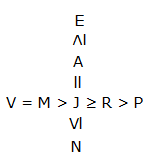I. A>P (True)

II. E<R (False)

III. M>E (True)

IV. R≥E (False)

Q.2) (e)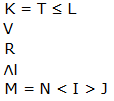I. T<N (False)

II. L>N (False)

III. K>J (False)

IV. L<M (False)

Q.3) (c)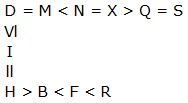I. I<Q (False)

II. S>R (False)

III. B>X (False)

IV. B<X (True)

Q.4) (e)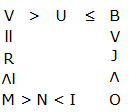I. U<N (False)

II. B>N (False)

III. V>J (False)

IV. B<M (False)

Q.5) (e)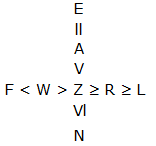I. A>L (True)

II. E<R (False)

III. W=E (False)

IV. R≥N (False)

Q.6) (d)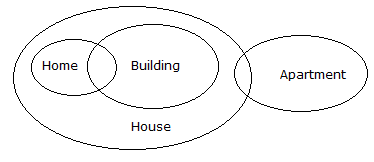I. False

II. False

III. False

Q.7) (c)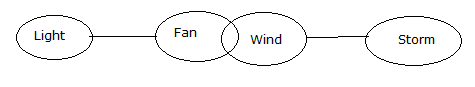I. False

II. True

III. True

Q.8) (c)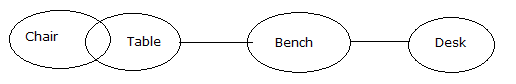I. False

II. False (either ‘or’)

III. False (either ‘or’)

Q.9) (a)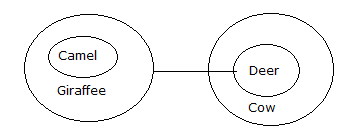I. True

II. True

III. False

Q.10) (b)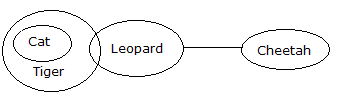I. True

II. False

III. False

Q.11) (d)

Symbol number consonant

> 0 M

* 3 H

\$ 8 V

Q.12) (c)

7 + 6 = 13th – (M) (Without numbers)

Q.13) (d)

Except d, all follows the same logic

Eg) C#J

-C#1st from the left end and 2nd from the left end

-J1st from the right end

But in option d,

N>11th and 12th element from the left end

I10th from the right end

Q.14) (e)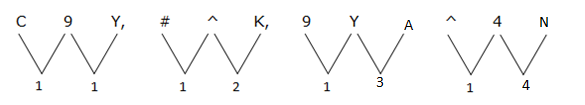Q.15) (b)

C # 9 ^ Y 4 K & A 6 N > 0 M 1 E * 3 H 2 L @ I 5 X \$ 8 V 7 % O J

F # 9 ^ B 4 N & Y 6 Q > 0 P 1 C * 3 K 2 O @ G 5 A \$ 8 Y 7 % M M

D.16-17)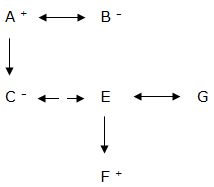Q.16) (e)

Q.17) (a)

Q.18) (b)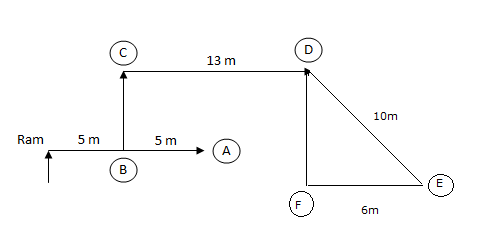Q.19)

dQ.20) (e)We will post this Practice Questions – Daily till the start of the IBPS RRB PO & Assistant (Regularly)

Quantitative Aptitude @ 3:00 P.M

Reasoning Ability -@ 5:00 P.M

 QUANTITATIVE APTITUDE REASONING DAY 1 DAY 1 DAY 2 DAY 2 DAY 3 DAY 3 DAY 4 DAY 4 DAY 5 DAY 5 DAY 6 DAY 6 DAY 7 DAY 7 DAY 8 DAY 8 DAY 9 DAY 9 DAY 10 DAY 10 DAY 11 DAY 12 DAY 12 DAY 13 DAY 13 DAY 14 DAY 14

Attend GEEK TEST – SOLVE 10 Daily and enhance your preparation to the next level. Attend the Daily Tests with the Timer and improve your solving ability.

Also Please attend this BLUE WHALE QUESTION (which is a combination of many topics and combined into a single one). This is a One Stop Solution for all your Miscellaneous needs.

## BLUE WHALE CHALLENGE QUESTIONS

Get the FULL LENGTH MOCK TEST SERIES for SBI PO Mains 2018 , SBI CLERK Mains 2018 & BOB PO Mains 2018 from our Bankersdaily Store.